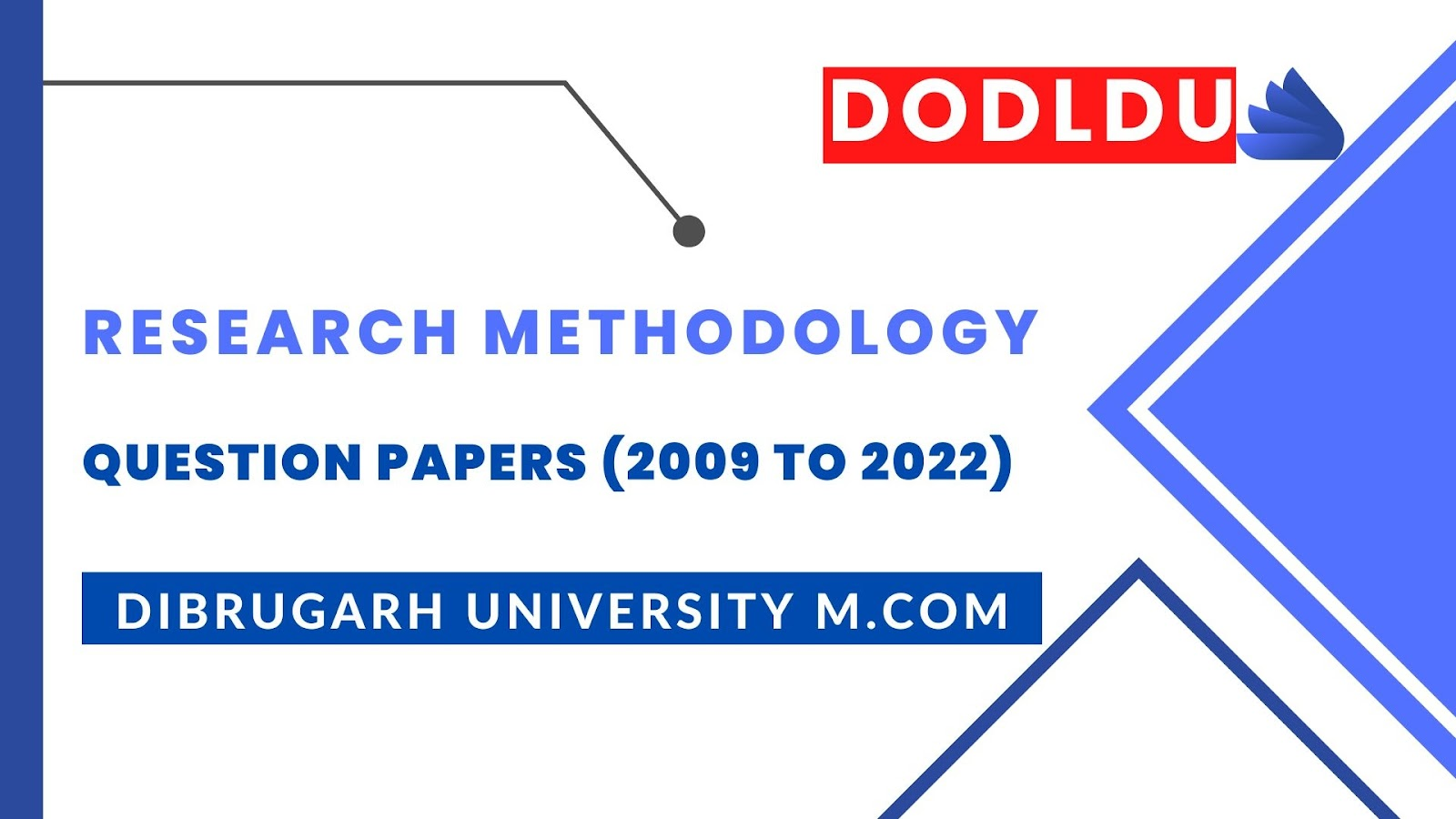# Research Methodology Question Paper 2016 [Dibrugarh University DODLDU M.Com Final Year]

## Research Methodology Question Paper 2016 (Nov-Dec)[Dibrugarh University DODLDU M.Com Final Year]

COMMERCE
Paper: 203
(Research Methodology and Statistical Analysis)
Full Marks: 80
Time: 3 hours
The figures in the margin indicate full marks for the questions
1. (a) What is Business Research? What factors should be taken into consideration while selecting a sample from a population?                         6+10=16
Or
(b) Detail out the measurement of scaling techniques.   16

2. (a) How does regression enable prediction of relationship between variables?         16
Or
(b) Explain the relationship between mean, median and mode.                 16
3. (a) State the importance of probability rules in research.                16
Or
(b) State the importance of probability distribution in research.             16

4. (a) What are the errors in hypothesis testing? State the general procedure for testing a hypothesis.   10+6=16
Or
(b) Define large and small sampling tests (Parametric).   16

5. (a) What are the assumptions underlying the use of x2 test? Also explain Chi-square test for goodness of fit.                  6+10
Or
(b) The scores of 7 boys and 8 girls students in a physical test is given below. Use the median test to find out whether the test scores differ significantly in the two sexes:      16
 Boys: 22 25 27 40 42 33 28 Girls: 20 32 34 31 39 29 28 33

***